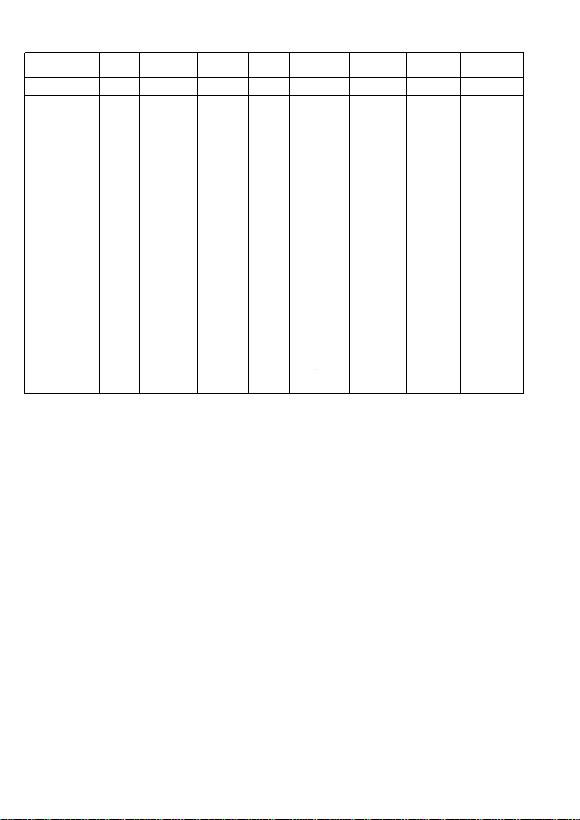Series Code Size
Code Tolerance
Code Packing
Code TC
Code Voltage
Code Termination
Code Process
Code Capacitance
Code
CC 0402 K R X7R 8 B B 102
CC = MLCC
CA = 4 x
capacitor array
CL = Low
inductance
CH = High
frequency
SC = Safety
certification
AC =
Automotive
CS = Soft
termination
0201
0402
0603
0805
1206
1210
1808
1812
0306
0508
0612
B= ± 0.1 pF
C= ± 0.25
pF
D= ± 0.5 pF
F = ± 1%
G = ± 2%
J = ± 5%
K = ± 10%
M = ± 20%
Z = -20% to
+80%
R = 7"
Paper/PE
tape reel
P = 13"
Paper/PE
tape reel
K = 7"
plastic
tape reel
F = 13"
plastic
tape reel
C = Bulk
case
NP0
X5R
X7R
Y5V
5 = 6.3 V
6 = 10 V
7 = 16 V
8 = 25 V
9 = 50 V
0 = 100 V
A = 200 V
B = 500 V
C = 1kV
D = 2kV
E = 3kV
G = 35 V
S = 2.5 kV
T = X2/Y3
for TUV/UL
(3 kV)
W = X1/Y2
for TUV/UL
(4 kV)
U = X1/Y2
for TUV/UL
(5 kV)
Y = 250
V
B = Ni-
Barrier N - NP0
B = Class 2
products
0 = x 1
1 = x 10
1
2 = x 10
2
3 = x 10
3
4 = x 10
4
5 = x 10
5
6 = x 10
6
7 = x 10
7
R47 = 0.47
pF
4R7 = 4.7 pF
101 = 100 pF
104 = 0.1 uF
105 = 1 uF
226 = 22 uF
Y
250
V
Z = 630 V